Thermodynamics and Propulsion

# 12.2 Conservation of Angular Momentum

[This section is excerpted from Fluid Flow: A First Course in Fluid Mechanics, Macmillan Publishing Company, 1989.]

The momentum theorem developed in Chapter 10 gives the force acting on a fixed volume in terms of linear momentum flux through the surface of the volume. In many situations we are interested in the moment or torque on the volume. For this purpose we may adapt the angular momentum law of mechanics to the flow of fluids. Our starting point is the familiar lawwhere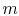,, andrefer to a single particle. The torque exerted by the forceabout a fixed point iswhere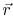is the radius vector from the fixed point to the point of application of. The symbol,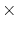, signifies, as usual, that the vector cross-product shall be taken. Then, from Newton's law of motion,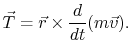We now define a vector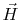as the vector product of the radius vector to the particle and the linear momentum, that is,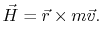The quantityis called angular momentum. Upon differentiatingwith respect to time, we find thatHowever,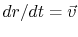and the cross-product of a vector parallel to itself is zero. The first term in the right-hand side therefore vanishes and we have the result that(12..1)

Equation (12.1) states that the rate of change of angular momentum of a particle about a fixed point is equal to the torque applied to the particle.

We now seek to modify the law as expressed by Equation (12.1) to be suitable for a fixed volume. The torque on a material volume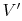is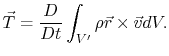This is readily transformed into a control volume integral. We have, therefore,where(12..2)

is the angular momentum contained within the control volume. Equation (12.2) represents the angular momentum theorem. [For more information about angular momentum and rotational energy, see pages 246 and 558 in Hibbeler's Engineering Dynamics.]

UnifiedTP